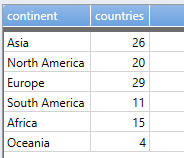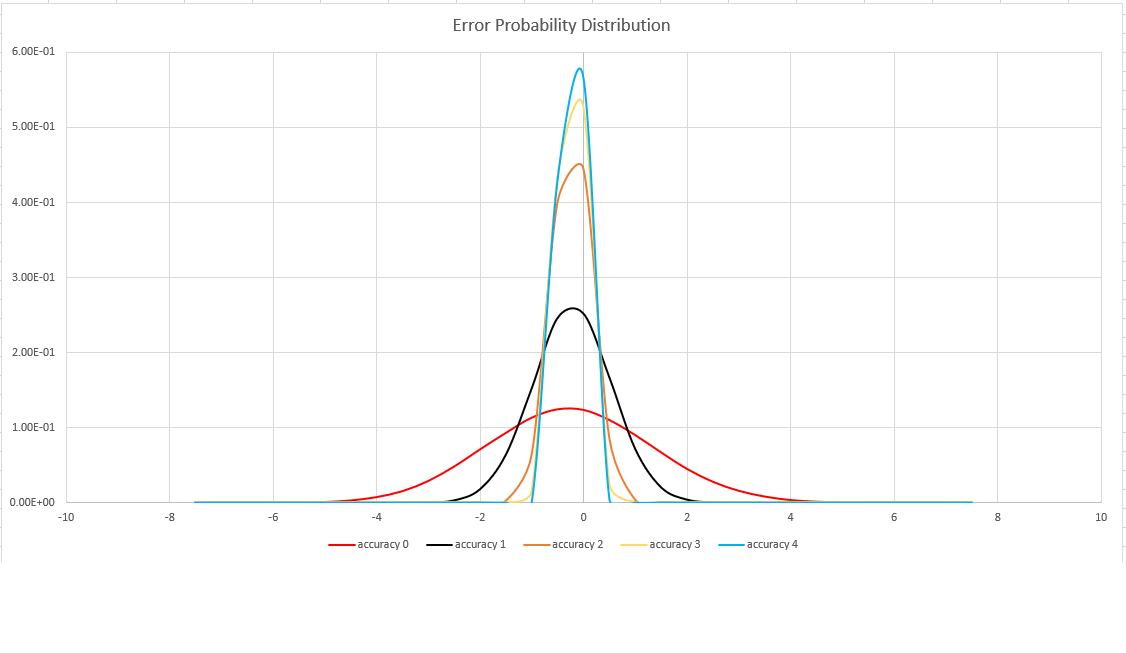# dcount() (aggregation function)

Returns an estimate for the number of distinct values that are taken by a scalar expression in the summary group.

Note

The `dcount()` aggregation function is primarily useful for estimating the cardinality of huge sets. It trades performance for accuracy, and may return a result that varies between executions. The order of inputs may have an effect on its output.

## Syntax

`dcount` `(`Expr[`,` Accuracy]`)`

## Arguments

• Expr: A scalar expression whose distinct values are to be counted.
• Accuracy: An optional `int` literal that defines the requested estimation accuracy. See below for supported values. If unspecified, the default value `1` is used.

## Returns

Returns an estimate of the number of distinct values of `Expr` in the group.

## Example

``````PageViewLog | summarize countries=dcount(country) by continent
``````Get an exact count of distinct values of `V` grouped by `G`.

``````T | summarize by V, G | summarize count() by G
``````

This calculation requires a great amount of internal memory, since distinct values of `V` are multiplied by the number of distinct values of `G`. It may result in memory errors or large execution times. `dcount()`provides a fast and reliable alternative:

``````T | summarize dcount(V) by G | count
``````

## Estimation accuracy

The `dcount()` aggregate function uses a variant of the HyperLogLog (HLL) algorithm, which does a stochastic estimation of set cardinality. The algorithm provides a "knob" that can be used to balance accuracy and execution time per memory size:

Accuracy Error (%) Entry count
0 1.6 212
1 0.8 214
2 0.4 216
3 0.28 217
4 0.2 218

Note

The "entry count" column is the number of 1-byte counters in the HLL implementation.

The algorithm includes some provisions for doing a perfect count (zero error), if the set cardinality is small enough:

• When the accuracy level is `1`, 1000 values are returned
• When the accuracy level is `2`, 8000 values are returned

The error bound is probabilistic, not a theoretical bound. The value is the standard deviation of error distribution (the sigma), and 99.7% of the estimations will have a relative error of under 3 x sigma.

The following image shows the probability distribution function of the relative estimation error, in percentages, for all supported accuracy settings: